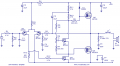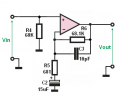# Questions about the operation of a 50W audio amplifier

#### janjan22

Joined Jun 10, 2015
14
Hi all,

I have some questions about the operation of a 50W amplifier attached as an image.

1. Which path follows the DC base current of Q2 (input pair)? For Q1 there is a DC patch through the input source and 47K resistor, but for Q2 it doesn't look so obvious where the DC base current is going. I think the only possibility is through the load?

2. What's the purpose of R12? In other audio designs it seems that sometimes this resistor is not used.

3. It is said that the output signal of an input pair is a current signal (transconductance stage). But in this schematic the base of Q4 (VAS transistor) is connected through R12 to the collector output voltage of Q1. So it seems it is voltage driven, because it is connected to the collector output voltage. Then why it is said that the output signal of the input pair is a current signal?

I have some other questions, but I'll save them for later. Thanks in advance.#### Jony130

Joined Feb 17, 2009
5,445
Q5 provide a path for Q2 base current.

Ask the designers, in this case IRF designed this amp.
http://www.irf.com/technical-info/appnotes/an-948.pdf
I do not see any compensation capacitor, so maybe they add such a "big" resistor in series with VAS base for stabilization purpose.
Or maybe they want to reduce the influence of a Q4 hie (rin) variation. Because hie is a load for a LTP (long tail pair).

But in this case the "current" from LTP is the signal. So how can R12 has any effect on the Q4 base current?

#### Jony130

Joined Feb 17, 2009
5,445
Oscillation of the amplifier caused by capacitive coupling to the base of the driver transistor, Q4, is suppressed by the addition of a series resistor, R14 (page 4)

#### janjan22

Joined Jun 10, 2015
14
Thanks for your fast response. That PDF is very useful. I didn't know it was a design from IRF. Coming back to the questions:

1. You say there is a path for the DC bias current through Q5, does that mean Q5 is always (slightly conducting)?

2. Thanks, that's more clear now.

3. Ok, I was assuming the base of Q4 is voltage driven because it is connected to the output collector voltage of Q1. Do you mean to say that R12 has no influence on the base current of Q4? So that the base current would be equal regardless of R12 included or not?

4. It is said in the IRF app. note that the bias current is said by R11 and R10 (In the PDF R8 and R9).
Quote "The class A driver transistor, Q4, operates at a bias current determined by resistors R8, R9, nominally 5mA."
But the bias of a transistor is always set at the base right? The base current sets the collector current and the collector load determines the collector output voltage. Then why they say it is said by R8 and R9?

#### Jony130

Joined Feb 17, 2009
5,445
1. You say there is a path for the DC bias current through Q5, does that mean Q5 is always (slightly conducting)
Yes, the P-MOS provide a path for Q2 base current. Also notice that this base current is very small Ic2 ≈ (35V - 0.65V)/(15kΩ + 1.2kΩ) * 0.5 ≈ 1mA and the base current is around 1mA/hfe = 10μA range.

3. Ok, I was assuming the base of Q4 is voltage driven because it is connected to the output collector voltage of Q1. Do you mean to say that R12 has no influence on the base current of Q4? So that the base current would be equal regardless of R12 included or not?
I was talking about a "signal" current , not DC quiescent current. R12 is in series with Q4 base and thanks to this IR12 = IbQ4.
And R12 will have a small effect on the Ib4. We can easily neglect it.
R12 job is to form a low pass filter together with Cbse and Q4 miller capacitance and kept the amp stable.

4. It is said in the IRF app. note that the bias current is said by R11 and R10 (In the PDF R8 and R9).
Quote "The class A driver transistor, Q4, operates at a bias current determined by resistors R8, R9, nominally 5mA."
But the bias of a transistor is always set at the base right? The base current sets the collector current and the collector load determines the collector output voltage. Then why they say it is said by R8 and R9?
And they are right. In this type of a circuit the negative feedback loop help as set the DC bias point in the circuit, we have exactly the same situation as in the op amp, in fact this circuit is just a big and powerful op amp.
And because of this (without any input signal) the output voltage is around 0V thanks to negative feedback and differential amp (Q1 , Q2).
So, the voltage at Q4 collector is Vout - Vgs and at bottom of a R10 is Vout + Vgs. And from there we can find Ic4 as a:
Ic4 ≈ (35V - 4V)/(R10+R11) ≈ 5.7mA

Last edited:

#### MikeML

Joined Oct 2, 2009
5,444
... I didn't know it was a design from IRF. ...
The owners of CircuitsToday steal from everybody without attribution...

#### GopherT

Joined Nov 23, 2012
8,009
The owners of CircuitsToday steal from everybody without attribution...
Agreed. They can't even copy and paste correctly on some topics - humorous to me but sad to anyone trying to build a project based on their instructions.

#### janjan22

Joined Jun 10, 2015
14
I'm going to read this other threads and probably come back after the weekend. It seems there is more to this subject then appears from the surfaceYes, the P-MOS provide a path for Q2 base current. Also notice that this base current is very small Ic2 ≈ (35V - 0.65V)/(15kΩ + 1.2kΩ) * 0.5 ≈ 1mA and the base current is around 1mA/hfe = 10μA range.
1. To calculate Ic2 you assumed that the base voltage of Q2 is at 0V? I don't see how this is at 0V.

2.Is the base of Q1 also at 0V?

3.
And from there we can find Ic4 as a:
Ic4 ≈ (35V - 4V)/(R10+R11) ≈ 5.7mA
Where does the 4V come from in this calculation?

Thanks

#### Jony130

Joined Feb 17, 2009
5,445
1. To calculate Ic2 you assumed that the base voltage of Q2 is at 0V? I don't see how this is at 0V.
2.Is the base of Q1 also at 0V?
Yes, I assumed that Q1 and Q2 base is at 0V (without input signal of course). And this is a very reasonable assumption in real life base voltage is not larger than 0.6V or I should say it should be less than 0.6V.
You do not know why it is so? Try answer this question:
What is the voltage at Vin an Vout for this circuit (without input signal)Where does the 4V come from in this calculation?
N-MOS Vgs voltage. Again this is an assumption because it is impossible to know the exact value. And this is also the reason why nobody makes exact calculations in this type of a circuit, too many unknowns. And in real circuit this is not the most important part.

#### janjan22

Joined Jun 10, 2015
14
That opamp example makes it more clear. Vout should be 0V when input is also 0V. My confusion remains with the bias curent of Q4 VAS transistor.

In transistor theory it is always learned that the collector current is determined by the Vbe voltage (Ebers-Moll model) or input current (current model).
This collector current will flow regardless of load dimension this current will flow (within compliance). When there wouldn't be any signal at the base of Q4 there would be no collector current. I understand your calculation "Ic4 ≈ (35V - 4V)/(R10+R11) ≈ 5.7mA", but at the same time the bias current through Q4 must be set by an input signal at the base right?

#### Jony130

Joined Feb 17, 2009
5,445
Do you understand how negative feedback work?

As we establish for Vin = 0V we have Vout= 0V thanks to negative feedback action. And because of this output voltage will finally reach 0V.
This mines that Q4 is driven by Q1 (voltage drop across R13) in such a way to achieve this goal (Vout = 0V). And when circuit finally "set" vout at 0V the Q4 quiescent current must be equal to Ic4 ≈ (35V - 4V)/(R10+R11) ≈ 5.7mA.
If for example we lower R10 and R11 value (2K). The voltage at Q4 collector will rise from 4V to Vc4 = 35V - 4kΩ*5.7mA = 12.2V.
This rise in Q4 collector will appears as a Vout voltage rise from 0V to 8.2V. This increase in output voltage will reduce Q2 Vbe voltage and Q2 will reduce his collector current. So more current from R3 can now flows into Q1.
Q1 collector will increase --->more voltage drop across R13---> large Q4 Vbe---> Q4 collector current will increase his value---> large voltage drop across R10+R11---> Vc4 will drop-->Vout will decrease. And circuit will stop all this action when Vout = 0V. And IcQ4 will have a new value Ic = (35V - 4V)/4kΩ = 7.75mA
I hope you finally "see" how R10+R11 "sets" the Q4 quiescent current, and all this is possible thanks to negative feedback.

•janjan22